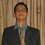# JEE mechanics doubt!! plz help

Q. If angular velocity of a disc depends on angle rotated $$\theta$$ as $$\omega$$ =$$\theta^{2}$$ +$$2\theta$$, then its angular acceleration $$\alpha$$ at $$\theta$$=1 rad is

(1) 8 rad/$sec^{2}$
(2) 10 rad/$sec^{2}$
(3) 12 rad/$sec^{2}$
(4) None of theseNote by Akhilesh Chobey
7 years, 1 month ago

This discussion board is a place to discuss our Daily Challenges and the math and science related to those challenges. Explanations are more than just a solution — they should explain the steps and thinking strategies that you used to obtain the solution. Comments should further the discussion of math and science.

When posting on Brilliant:

• Use the emojis to react to an explanation, whether you're congratulating a job well done , or just really confused .
• Ask specific questions about the challenge or the steps in somebody's explanation. Well-posed questions can add a lot to the discussion, but posting "I don't understand!" doesn't help anyone.
• Try to contribute something new to the discussion, whether it is an extension, generalization or other idea related to the challenge.

MarkdownAppears as
*italics* or _italics_ italics
**bold** or __bold__ bold
- bulleted- list
• bulleted
• list
1. numbered2. list
1. numbered
2. list
Note: you must add a full line of space before and after lists for them to show up correctly
paragraph 1paragraph 2

paragraph 1

paragraph 2

[example link](https://brilliant.org)example link
> This is a quote
This is a quote
    # I indented these lines
# 4 spaces, and now they show
# up as a code block.

print "hello world"
# I indented these lines
# 4 spaces, and now they show
# up as a code block.

print "hello world"
MathAppears as
Remember to wrap math in $$ ... $$ or $ ... $ to ensure proper formatting.
2 \times 3 $2 \times 3$
2^{34} $2^{34}$
a_{i-1} $a_{i-1}$
\frac{2}{3} $\frac{2}{3}$
\sqrt{2} $\sqrt{2}$
\sum_{i=1}^3 $\sum_{i=1}^3$
\sin \theta $\sin \theta$
\boxed{123} $\boxed{123}$

Sort by:

First differentiate omega with respect to t to get the expression of alpha then put the value of omega at theta 1 and theta

- 6 years, 9 months ago

i think angular acc is dw/dt to find that : first find dw/dtheta and then as we know w = dtheta/dt

so now take out inverse of w which is dt/dtheta and now divide dw/dtheta and dt/dtheta

- 6 years ago

since a(alpha) = dw/dt = (dw/ds )(ds/dt)=w(dw/ds).Hence ans will be 3*4=12

- 5 years, 10 months ago